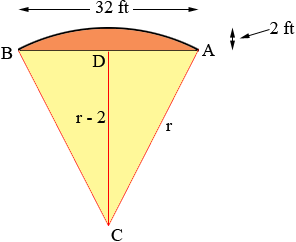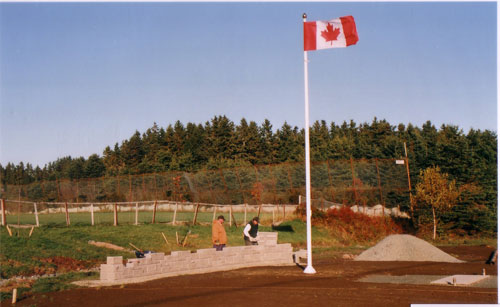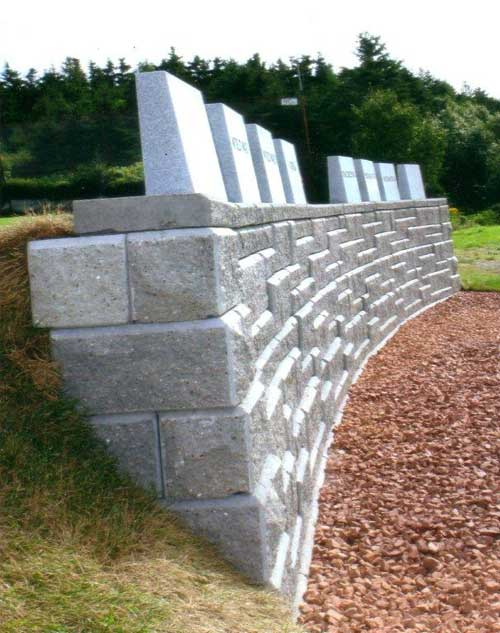SEARCH HOMEMath Central Quandaries & QueriesQuestion from Don, a parent: Thank you for such a great site. Unfortunately the depth of my math skills leaves me unable to figure out this question. I am trying to determine the length of an arch with a two foot backset from 32 foot frontage. The answer to this question will permit us to carry on with the construction of our veterans memorial wall. Thank You DonHi Don,

Here is how I am interpreting your question. Please let me know if my interpretation is incorrect.You have a 32 foot frontage, (A to B in my diagram) and a circular arc from A to B that is backset 2 feet at the midpoint of the frontage, D in my diagram. I let C be the centre of the circle and called its radius r feet. ADC is a right triangle with |AD| = 16 feet and |DC| = r - 2 feet and hence using Pythagoras theorem

r2 = (r - 2)2 + 162 = r2 - 4r + 4 + 256

Thus

4r = 260

and hence

r = 65 feet.

If the angle BCA is measured in radians then the length of the arc from A to B is

arclength = r × angle BCA

I can find the angle DCA which is half of the angle BCA because tan(DCA) = |AD|/|DC| = 16/63 = 0.2540. Thus angle DCA = tan-1(0.2540) = 0.2487 radians and angle BCA = 2 × 0.2487 = 0.4974 radians. Hence

arclength = r × angle BCA = 65 × 0.4974 = 32.33 feet.

Harley

In August 2009 Don sent us these photos of the veterans memorial wallMath Central is supported by the University of Regina and The Pacific Institute for the Mathematical Sciences.